# Methods to Rank Rows in SQL Server: ROW_NUMBER(), RANK(), DENSE_RANK() and NTILE()

Total: 58 Average: 4.3

SQL Server provides us with a number of window functions that help us to perform calculations across a set of rows, without the need to repeat the calls to the database. Unlike the standard aggregate functions, the window functions will not group the rows into a single output row, they will return a single aggregated value for each row, keeping the separate identities for those rows. The Window term here is not related to the Microsoft Windows operating system, it describes the set of rows that the function will process.

One of the most useful types of window functions is Ranking Window Functions that are used to rank specific field values and categorize them according to the rank of each row, resulting in a single aggregated value for each participated row. There are four ranking window functions supported in SQL Server; ROW_NUMBER(), RANK(), DENSE_RANK() and NTILE(). All these functions are used to calculate ROWID for the provided rows window in their own way.

Four ranking window functions use the OVER() clause that defines a user-specified set of rows within a query result set. By defining the OVER() clause, you can also include the PARTITION BY clause that determines the set of rows the window function will process, by providing column or comma separated columns to define the partition. In addition, the ORDER BY clause can be included, which defines the sorting criteria within the partitions that the function will go through the rows while processing.

In this article, we will discuss how to use four ranking window functions: ROW_NUMBER(), RANK(), DENSE_RANK() and NTILE() practically, and the difference between them.

To serve our demo, we will create a new simple table and insert few records into the table using the T-SQL script below:

You can check that the data is inserted successfully using the following SELECT statement:

With the sorted result applied, the result set is as follows below: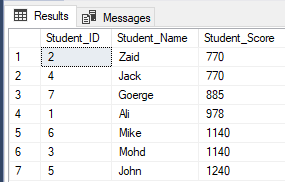### ROW_NUMBER()

The ROW_NUMBER() ranking window function returns a unique sequential number for each row within the partition of the specified window, starting at 1 for the first row in each partition and without repeating or skipping numbers in the ranking result of each partition. If there are duplicate values within the row set, the ranking ID numbers will be assigned arbitrarily. If the PARTITION BY clause is specified, the ranking row number will be reset for each partition. In the previously created table, the query below shows how to use the ROW_NUMBER ranking window function to rank the StudentScore table rows according to the score of each student:

It is clear from the result set below that the ROW_NUMBER window function ranks the table rows according to the Student_Score column values for each row, by generating a unique number of each row that reflects its Student_Score ranking starting from the number 1 without duplicates or gaps and dealing with all the rows as one partition. You can see also that the duplicate scores are assigned to different ranks randomly:If we modify the previous query by including the PARTITION BY clause to have more than one partition, as shown in the T-SQL query below:

The result will show that the ROW_NUMBER window function will rank the table rows according to the Student_Score column values for each row, but it will deal with the rows that have the same Student_Score value as one partition. You will see that a unique number will be generated for each row reflecting its Student_Score ranking, starting from the number 1 without duplicates or gaps within the same partition, resetting the rank number when moving to a different Student_Score value.

For example, the students with score 770 will be ranked within that score by assigning a rank number to it. However, when it is moved to the student with score 885, the rank starting number will be reset to start again at 1, as shown below: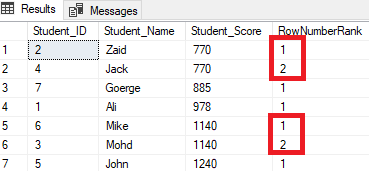### RANK()

The RANK() ranking window function returns a unique rank number for each distinct row within the partition according to a specified column value, starting at 1 for the first row in each partition, with the same rank for duplicate values and leaving gaps between the ranks; this gap appears in the sequence after the duplicate values. In other words, the RANK() ranking window function behaves like the ROW_NUMBER() function except for the rows with equal values, where it will rank with the same rank ID and generate a gap after it. If we modify the previous ranking query to use the RANK() ranking function:

You will see from the result that the RANK window function will rank the table rows according to the Student_Score column values for each row, with a ranking value reflecting its Student_Score starting from the number 1, and ranking the rows that have the same Student_Score with the same rank value. You can also see that two rows having Student_Score equal to 770 are ranked with the same value, leaving a gap, which is the missed number 2, after the second-ranked row. The same happens with the rows where Student_Score equals 1140 that are ranked with the same value, leaving a gap, which is the missing number 6, after the second row, as shown below:Modifying the previous query by including the PARTITION BY clause to have more than one partition, as shown in the T-SQL query below:

The ranking result will have no meaning, as the rank will be done according to Student_Score values per each partition, and the data will be partitioned according to the Student_Score values. And due to the fact that each partition will have rows with the same Student_Score values, the rows with the same Student_Score  values in the same partition will be ranked with the value equal to 1. Thus, when moving to the second partition, the rank will be reset, starting again with the number 1, having all ranking values equal to 1 as shown below: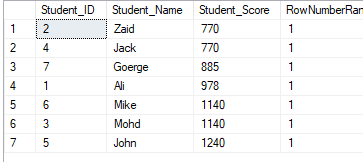### DENSE_RANK()

The DENSE_RANK() ranking window function is similar to the RANK() function by generating a unique rank number for each distinct row within the partition according to a specified column value, starting at 1 for the first row in each partition, ranking the rows with equal values with the same rank number, except that it does not skip any rank, leaving no gaps between the ranks.

If we rewrite the previous ranking query to use the DENSE_RANK() ranking function:Again, modify the previous query by including the PARTITION BY clause to have more than one partition, as shown in the T-SQL query below:

The ranking values will have no meaning, where all the rows will be ranked with the value 1, due to assigning the duplicate values to the same ranking value and resetting the rank starting id when processing a new partition, as shown below: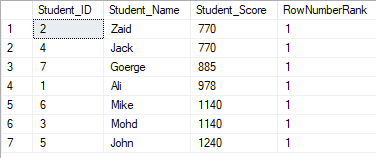### NTILE(N)

The NTILE(N) ranking window function is used to distribute the rows in the rows set into a specified number of groups, providing each row in the row set with a unique group number, starting with the number 1 that shows the group this row belongs to, where N is a positive number, which defines the number of groups you need to distribute the rows set into.

In other words, if you need to divide specific data rows of the table into 3 groups, based on particular column values, the NTILE(3) ranking window function will help you to achieve this easily.

The number of rows in each group can be calculated by dividing the number of rows into the required number of groups. If we modify the previous ranking query to use NTILE(4) ranking window function to rank seven table rows into four groups as the T-SQL query below:

The number of rows should be (7/4=1.75) rows into each group. Using the NTILE() function, SQL Server Engine will assign 2 rows to the first three groups and one row to the last group, in order to have all the rows included into the groups, as shown in the result set below: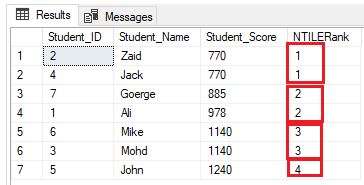Modifying the previous query by including the PARTITION BY clause to have more than one partition, as shown in the T-SQL query below:

The rows will be distributed into four groups on each partition. For example, the first two rows with Student_Score equal to 770 will be in the same partition, and will be distributed within the groups ranking each one with a unique number, as shown in the result set below: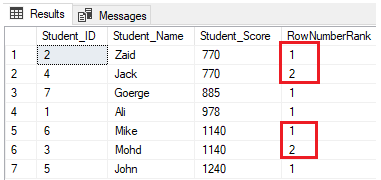### Putting All Together

To have a more clear comparison scenario, let us truncate the previous table, add another classification criterion, which is the class of the students, and finally insert new seven rows using the T-SQL script below:

After that, we will rank seven rows according to each student score, partitioning the students according to their class. In other words, each partition will include one class, and each class of students will be ranked according to their scores within the same class, using four previously described ranking window functions, as shown in the T-SQL script below:

Due to the fact that there are no duplicate values, four ranking window functions will work in the same way, returning the same result, as shown in the result set below: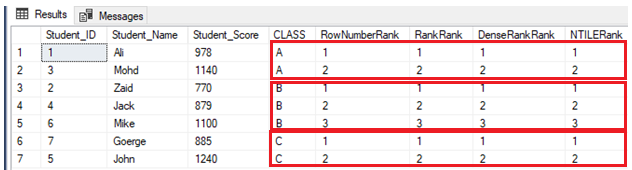If another student is included in class A with a score, that another student in the same class already has, using the INSERT statement below:

Nothing will change for the ROW_NUMBER() and NTILE() ranking window functions. The RANK and DENSE_RANK() functions will assign the same rank for the students with the same score, with a gap in the ranks after the duplicate ranks when using the RANK function and no gap in the ranks after the duplicate ranks when using the DENSE_RANK(), as shown in the result below: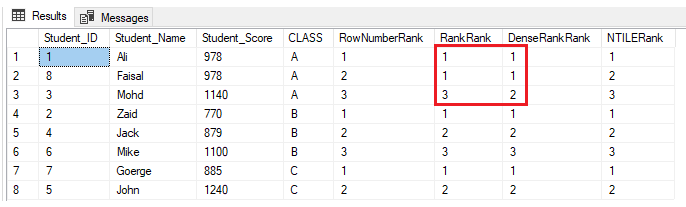### Practical Scenario

The ranking window functions are used widely by SQL Server developers. One of the common scenarios for the ranking functions usage, when you want to fetch specific rows and skip others, using the ROW_NUMBER(,) ranking window function within a CTE, as in the T-SQL script below that returns the students with ranks between 2 and 5 and skip the others:

The result will show that only students with ranks between 2 and 5 will be returned: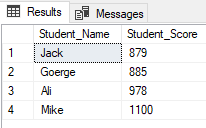Starting from SQL Server 2012, a new useful command, OFFSET FETCH was introduced that can be used to perform the same previous task by fetching specific records and skipping the others, using the T-SQL script below:

Retrieving the same previous result as shown below: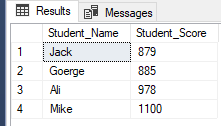### Conclusion

SQL Server provides us with four ranking window functions that help us to rank the provided rows set according to specific column values. These functions are: ROW_NUMBER(), RANK(), DENSE_RANK() and NTILE(). All these ranking functions perform the ranking task in its own way, returning the same result when there are no duplicate values in the rows. If there is a duplicate value within the row set, the RANK function will assign the same ranking ID for all rows with the same value, leaving gaps between the ranks after the duplicates. The DENSE_RANK function will also assign the same ranking ID for all rows with the same value, but will not leave any gap between the ranks after the duplicates. We go through different scenarios within this article to cover all possible cases that help you to understand the ranking window functions practically.

### References: